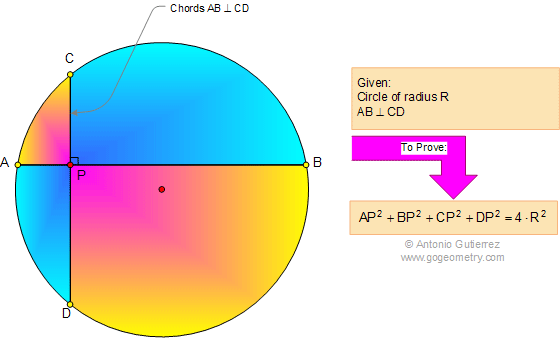# Geometry Problem 651: Archimedes' Book of Lemmas: Proposition 11 - Perpendicular Chords and Radius

If two chords AB, CD in a circle of radius R intersect at right angles in a point P, not being the center,then AP2 + BP2 + CP2 + DP2 = 4.R2.## Books of Lemmas Index